﻿ Logical Circuits and Their Applications

### Logical Circuits and Their Applications

Ľubica Miková, Michal Kelemen, Alexander Gmiterko, Lukáš Kačmár

Journal of Automation and Control

## Logical Circuits and Their Applications

Ľubica Miková1,, Michal Kelemen1, Alexander Gmiterko1, Lukáš Kačmár1

1Technical University of Košice, Faculty of Mechanical Engineering, Department of Mechatronics, Košice, Slovakia

### Abstract

Logic circuits are inseparable part of almost every modern electronic device. Due to constant raising of requirements of society for technical complexity of these devices, complex integrated circuits are used more frequently. This article deals with mathematical apparatus, which logic circuits work on, and to explain methods of entry and execution of logical functions.

• Ľubica Miková, Michal Kelemen, Alexander Gmiterko, Lukáš Kačmár. Logical Circuits and Their Applications. Journal of Automation and Control. Vol. 3, No. 3, 2015, pp 106-109. http://pubs.sciepub.com/automation/3/3/13
• Miková, Ľubica, et al. "Logical Circuits and Their Applications." Journal of Automation and Control 3.3 (2015): 106-109.
• Miková, Ľ. , Kelemen, M. , Gmiterko, A. , & Kačmár, L. (2015). Logical Circuits and Their Applications. Journal of Automation and Control, 3(3), 106-109.
• Miková, Ľubica, Michal Kelemen, Alexander Gmiterko, and Lukáš Kačmár. "Logical Circuits and Their Applications." Journal of Automation and Control 3, no. 3 (2015): 106-109.

 Import into BibTeX Import into EndNote Import into RefMan Import into RefWorks

1234
Prev Next

### 1. Introduction

Logic circuits are part of nearly each electronic device we come in contact with everyday. Cell phone, laptop or plasma television, all these devices contain within complicated, yet compact integrated circuits, which are however made of basic, simple logical circuits.

### 2. Logics

There are circuits which can be defined by continuous time courses. These are mainly constant, linear and occasionally even nonlinear courses. A good example is sinusoidal that possibly contains a phase shift and is dependent on circuit type. These courses are called analog signals [1, 2, 3].Download asVeiw figureFigures index
Figure 1. Examples of analog signals 

In the process of electronics development there was an improvement of electronic devices, inside which components operating on the principle of numerical methods are nowadays more and more used. Numerical circuits are characterized by discontinuous (step) time change – numerical or digital .Download asVeiw figureFigures index
Figure 2. Digital signal 

### 3. Basic Division of Logic Circuits

Logic circuits can be divided according to a few aspects:

According to the logic function they realize

- combinational – output variable depends only on input variables in a specific point of time,

- sequential – output variable is dependent not only on input variables in a specific point of time, but also on the sequence of input variables in previous points of time .

According to their ability to amplify the signal

- passive,

- active .

3.1. Analysis and Synthesis of Logic Circuits

All logic circuits are as cybernetic systems characterized by behavior defined by logic function, Karnaugh map or truth table and structure, which is expressed by schematics diagram of a logic circuit.

Given the initial impulse and the result of action we know two basic approaches:

- analysis – scrutiny of behavior and functioning of designed or already realized circuit,

- synthesis – method where, at defined initial conditions of system behavior and requirements on its control, we perform the design of a specific logic circuit that is realizing this behavior .Download asVeiw figureFigures index
Figure 3. Analysis and synthesis 

### 4. Logical Circuits

4.1. Combinational Logic Circuits

Combinational logic circuit (CLC) has its output state dependent on the immediate state of inputs. Value of output variables is defined only by immediate combination of input variables [4, 6].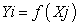where i= 1,2,...m, j=1,2,...n.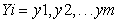– output variables combinational logic circuits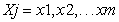– input variables combinational logic circuitsDownload asVeiw figureFigures index
Figure 4. Combinational logic circuits

CLC is realized as a functional whole by connection of logic members or as integrated circuit. Main representers of CLCs are:

- coders and decoders,

- multiplexers and demultiplexers,

- comparators,

- circuits performing arithmetic operations (adders and so on) .

4.2. Sequential Logic Circuits

Sequential logic circuit is defined by a circuit where the immediate value of outputs depends on the immediate values of inputs and also on states they were in previously. They contain memory parts for storing previous state of circuit .

Function of sequential logic circuits can be described by two equations:

1) Equation for outputs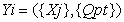2) Equation for following internal state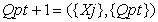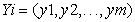- output set of sequential logic circuits- input set of sequential logic circuits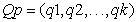- internal state set of sequential logic circuits.

So called internal variablescome from outputs of individual flip-flop circuits. Internal state consists of set of all outputs of flip-flop circuits in a specific time t. Index t discerns actual internal stateand index t+1 the following state of sequential circuits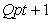.

Figure 5 illustrates block diagram of sequential logic circuits comprised of combinational and sequential part. The combinational part generates values of outputs and actuating signals of memory circuits. Sequential part contains memory circuits (usually flip-flop circuits), which are controlled by periodical numerical signal with period of T. .Download asVeiw figureFigures index
Figure 5. Block diagram of sequential logic circuit
4.3. Examples of Logic Control

One of possible examples of logic control is design of logic circuit for control of water pumping from a well.

Pump will operate if there is insufficient amount of water in the tank inside the house and sufficient amount of water in the well. If there is sufficiency of water in the tank inside the house and insufficiency of water in the well, yellow indicator light will light up. If there is insufficiency of water both in the tank inside the house and in the well, red indicator light will light up.Download asVeiw figureFigures index
Figure 6. Design of logic circuit for control of water pumping

After compiling a truth table and subsequently a Karnaugh map it is possible to create a design of control of this logic circuit using Matlab/Simulink program.Download asVeiw figureFigures index
Figure 7. Logic circuit in Matlab/Simulink

Another example can be design of building alarm device. Monitored are two windows on ground floor and entry door. System is designed so that alarm is sounded in the event of infiltration of the object by a stranger.Download asVeiw figureFigures index
Figure 8. Design of building alarm device

Design of control in Matlab/Simulink program is shown on Figure 9.Download asVeiw figureFigures index
Figure 9. Logic circuit in Matlab/Simulink

Another application of logic control is control of valve of reserve pipeline. Pumps A, B, C and D are connected to pipeline p1 and p2. It is necessary to design a combination control logic circuit for automatic control of valve of the backup pipeline p2, if pump flow rate exceeds the allowed flow rate of pipeline p1 – Q1= 7 l/s, where the pump flow rates are:

- QA = 2 l/s

- QB = 3 l/s

- QC = 3 l/s

- QD = 6 l/sDownload asVeiw figureFigures index
Figure 10. Logic circuit in Matlab/Simulink

Same as in previous examples of logic control, the logic control design diagram is shown on Figure 11.Download asVeiw figureFigures index
Figure 11. Logic circuit in Matlab/Simulink

### 5. Conclusion

In the recent years, reversible logic has emerged as a promising technology having its applications in low power CMOS, quantum computing, nano technology and optical computing. Reversible logic circuits provide less power dissipation as well as distinct output assignment for each distinct input [8, 9].

The aim of this article was to provide theoretical basis for logic circuits topic and create solution for specific given problems.

Solving of logic control design problems lies in compiling truth tables, which are then used to create Karnaugh maps. Utilizing the rules of handling these maps we put together the resulting logic functions. Logic circuit was created in MATLAB/ Simulink software. Correctness of solution was verified by multiple simulations with changing input parameters and monitoring accuracy of model operation. Simulated model meets all requirements and is easily and intuitively controlled.

### Acknowledgement

This contribution is a result of the project Slovak Grant Agency – project VEGA 1/0937/12 “Development of non-traditional experimental methods for mechanical and mechatronical systems”, and project KEGA 048TUKE-4/2014 “Increasing of knowledge base of students in area of application of embedded systems in mechatronic systems”.

### References

  Náter, E.: Elektronika pre 2. ročník odbor elektrotechnika. 41s. [online] [cit. 17.4.2015]. Dostupné na internete: http://www.spsehalova.sk/ver1/images/norhar/predmetove_komisie/skripta%20_ele_2.pdf.In article  Elektronika. [online] [cit. 18.4.2015]. Dostupné na internete: http://ostrovskeho.sk/ucivo/data/tvp-2/elektronika-1.cast.pdf.In article  Diviš, Z., Chmelíková, Z.,Zdrálek, J.: Logické obvody. Ostrava: Vysoká škola baňská – Technická univerzita Ostrava, 1999, 150s., ISBN 80-7078-653-1.In article PubMed  Tóth, Š.: Simulátor logických obvodov. Fakulta riadenia a informatiky, Žilinská univerzita v Žiline, 2008, 59s. [online] [cit. 18.4.2015]. Dostupné na internete: .In article  Putiš, M.: Kombinačné logické obvody. Elektrotechnická fakulta, Žilinská univerzita v Žiline, 2008, 65s. [online] [cit. 20.4.2015]. Dostupné na internete: .In article  Komparátory. [online] [cit. 23.4.2015]. Dostupné na internete: .In article  Béres, P.: Simulácia sekvenčných logických obvodov s využitím modulu Stateflow. Elektrotechnická fakulta, Žilinská univerzita v Žiline, 2008, 56s. [online] [cit. 24.4.2015]. Dostupné na internete: .In article  M. Mahapatro, S. K. Panda, J. Satpathy, M. Saheel: Design of Arithmetic Circuits Using Reversible Logic Gates and Power Dissipation Calculation, 2010 International Symposium on Electronic System Design.In article View Article  Vasudevan D P, Lala P K and Parkerson J P.: Online Testable Reversible Logic Circuit Design using NAND Blocks, Proceedings of the 19th IEEE International Symposium on Defect and Fault Tolerance in VLSI Systems, 2004.In article View Article  Ľ. Miková, F. Trebuňa, The application of simulation methods for modeling mechatronic systems, Acta Mechanica Slovaca. Vol. 16, 2012.In article